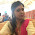### VHDL code for Basic Gates

AND Gate

library ieee;

use ieee.std_logic_1164.all;

entity and_gate is

port (a,b : in std_logic ;

c : out std_logic);

end and_gate;

architecture arc of and_gate is

begin

c <= a and b; end arc;

OR Gate

library ieee;

use ieee.std_logic_1164.all;

entity or_gate is

port (a,b : in std_logic ;

c : out std_logic);

end or_gate;

architecture arc of or_gate is

begin

c <= a or b; end arc;

NOT Gate

library ieee;

use ieee.std_logic_1164.all;

entity not_gate is

port (a: in std_logic ;

b : out std_logic);

end not_gate;

architecture arc of not_gate is

begin

b <= not a; end arc;

NAND Gate

library ieee;

use ieee.std_logic_1164.all;

entity nand_gate is

port (a,b : in std_logic ;

c : out std_logic);

end nand_gate;

architecture arc of nand_gate is

begin

c <= a or b; end arc;

NOR Gate

library ieee;

use ieee.std_logic_1164.all;

entity nor_gate is

port (a,b : in std_logic ;

c : out std_logic);

end nor_gate;

architecture arc of nor_gate is

begin

c <= a nor b; end arc;

XOR Gate

library ieee;

use ieee.std_logic_1164.all;

entity xor_gate is

port (a,b : in std_logic ;

c : out std_logic);

end xor_gate;

architecture arc of xor_gate is

begin

c <= a xor b;

end arc;

1.thank you so much for this blog. it was very useful to me!! i am new to vhdl and so it helped me a lot!!!!

2.3.nice one............

4.Thanx a lot, these are really helpful.
Do you have the codes for Multiplexer? 1 bit and 2bit?

1.entity counter is
Port ( clk,reset : in std_logic;
q : out std_logic_vector(1 downto 0));
end counter;

architecture Behavioral of counter is
type state is (st0,st1,st2,st3);
signal pstate,nstate:state;
begin
process(clk,reset)
begin
if(reset='1')then
pstate<=st0;
elsif(clk='1' and clk'event)then
pstate<=nstate;
else
null;
end if;
end process;
process(pstate)
begin
case pstate is
when st0=>
q<="00";
nstate<=st1;
when st1=>
q<="01";
nstate<=st2;
when st2=>
q<="10";
nstate<=st3;
when st3=>
q<="11";
nstate<=st0;
end case;
end process;

end Behavioral;

2.entity counter is
Port ( clk,reset : in std_logic;
q : out std_logic_vector(1 downto 0));
end counter;

architecture Behavioral of counter is
type state is (st0,st1,st2,st3);
signal pstate,nstate:state;
begin
process(clk,reset)
begin
if(reset='1')then
pstate<=st0;
elsif(clk='1' and clk'event)then
pstate<=nstate;
else
null;
end if;
end process;
process(pstate)
begin
case pstate is
when st0=>
q<="00";
nstate<=st1;
when st1=>
q<="01";
nstate<=st2;
when st2=>
q<="10";
nstate<=st3;
when st3=>
q<="11";
nstate<=st0;
end case;
end process;

end Behavioral;

3.kindly send me the code for PID controller...i'll be highly thankfull to you for this fovor

5.u r awwsum dude

6.2 good.....thanx

7.8.yeah it is good.. but please also mention architecture's type that it is solved in behaviour type or structural type or else..

9.thanks ...

10.need behavior codes pl...

11.thank you very much

12.Thank you :)